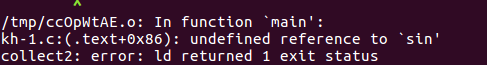• 编译错误： 解决方法： 将编译的语句 gcc hello.c -o hello.exe 改为 gcc hello.c -lm -o hello.exe 原因： sin函数定义在libm.so文件中。lm是libm的缩写。
编译错误：解决方法：
将编译的语句 gcc hello.c -o hello.exe 改为
gcc hello.c -lm -o hello.exe
原因：
sin函数定义在libm.so文件中。lm是libm的缩写。


展开全文linux
• C/C++ code/* @(#)k_sin.c 1.3 95/01/18 *//** ====================================================* Copyright (C) 1993 by Sun Microsystems, Inc. All rights reserved.** Developed at SunSoft, a Sun Micros...
C/C++ code/* @(#)k_sin.c 1.3 95/01/18 *//** ====================================================* Copyright (C) 1993 by Sun Microsystems, Inc. All rights reserved.** Developed at SunSoft, a Sun Microsystems, Inc. business.* Permission to use, copy, modify, and distribute this* software is freely granted, provided that this notice* is preserved.* ====================================================*/#ifndef lintstatic char rcsid[] = "$FreeBSD: src/lib/msun/src/k_sin.c,v 1.10 2005/11/02 13:06:49 bde Exp$";#endif/* __kernel_sin( x, y, iy)* kernel sin function on ~[-pi/4, pi/4] (except on -0), pi/4 ~ 0.7854* Input x is assumed to be bounded by ~pi/4 in magnitude.* Input y is the tail of x.* Input iy indicates whether y is 0. (if iy=0, y assume to be 0).** Algorithm*  1. Since sin(-x) = -sin(x), we need only to consider positive x.*  2. Callers must return sin(-0) = -0 without calling here since our*     odd polynomial is not evaluated in a way that preserves -0.*     Callers may do the optimization sin(x) ~ x for tiny x.*  3. sin(x) is approximated by a polynomial of degree 13 on*     [0,pi/4]*                   3            13*      sin(x) ~ x + S1*x + ... + S6*x*     where**  |sin(x)         2     4     6     8     10     12  |     -58*  |----- - (1+S1*x +S2*x +S3*x +S4*x +S5*x  +S6*x   )| <= 2*  |  x                               |**  4. sin(x+y) = sin(x) + sin'(x')*y*          ~ sin(x) + (1-x*x/2)*y*     For better accuracy, let*           3      2      2      2      2*      r = x *(S2+x *(S3+x *(S4+x *(S5+x *S6))))*     then                   3    2*      sin(x) = x + (S1*x + (x *(r-y/2)+y))*/#include "math.h"#include "math_private.h"static const doublehalf =  5.00000000000000000000e-01, /* 0x3FE00000, 0x00000000 */S1  = -1.66666666666666324348e-01, /* 0xBFC55555, 0x55555549 */S2  =  8.33333333332248946124e-03, /* 0x3F811111, 0x1110F8A6 */S3  = -1.98412698298579493134e-04, /* 0xBF2A01A0, 0x19C161D5 */S4  =  2.75573137070700676789e-06, /* 0x3EC71DE3, 0x57B1FE7D */S5  = -2.50507602534068634195e-08, /* 0xBE5AE5E6, 0x8A2B9CEB */S6  =  1.58969099521155010221e-10; /* 0x3DE5D93A, 0x5ACFD57C */double__kernel_sin(double x, double y, int iy){double z,r,v;z   =  x*x;v   =  z*x;r   =  S2+z*(S3+z*(S4+z*(S5+z*S6)));if(iy==0) return x+v*(S1+z*r);else      return x-((z*(half*y-v*r)-y)-v*S1);}
展开全文• 问题描述：C语言：利用函数指针编写一个用矩形法求定积分的通用函数,包括正弦,余弦和指数函数,//我运行的结果和答案对不上,请帮我看看程序对吗?//实现积分#include#includeint main(){float fsin(float);float fcos...
问题描述：C语言：利用函数指针编写一个用矩形法求定积分的通用函数,包括正弦,余弦和指数函数,//我运行的结果和答案对不上,请帮我看看程序对吗?//实现积分#include#includeint main(){float fsin(float);float fcos(float);float fexp(float);float fun(float,float,float(*)(float),int);float a1,b1,a2,b2,a3,b3;int n=20;float (*p)(float);printf("input a1,b1 for sin(x):\n");scanf("%f%f",&a1,&b1);printf("input a2,b2 for cos(x):\n");scanf("%f%f",&a2,&b2);printf("input a3,b3 for exp(x):\n");scanf("%f%f",&a3,&b3);p=fsin;printf("sin(x)的积分：%f\n",fun(a1,b1,p,n));p=fcos;printf("cos(x)的积分：%f\n",fun(a2,b2,p,n));p=fexp;printf("exp(x)的积分：%f\n",fun(a3,b3,p,n));getch();return 0;}float fsin(float x){return sin(x);}float fcos(float x){return cos(x);}float fexp(float x){return exp(x);}float fun(float a,float b,float(*p)(float x),int n){int i;float x,h,s;h=(b-a)/n;x=a;s=0;for(i=0;i1个回答分类：综合2014-12-07问题解答：我来补答for(i=0;i展开全文阅读
展开全文• 通过麦格劳林展开式，来编写一个sin函数C语言内置了三角函数，这个三角函数在 #include<math.h> 的头文件下要输入角度数，是有着需要先将角度转化为弧度在进行使用，我编写sin函数当精度太高时高角度数会...
通过麦格劳林展开式，来编写一个sin函数，C语言内置了三角函数，这个三角函数在   #include<math.h>  的头文件下要输入角度数，是有着需要先将角度转化为弧度在进行使用，我编写的sin函数当精度太高时高角度数会无法执行，可能存在数据溢出

​// sin.cpp : sinx=x-x*x*x/3*2+x*x*x*x*x/5*4*3*2-...

#include "stdafx.h"
#include"math.h"

double jiecheng (int n)
{
long sum=1;
while(n>1)
{
sum*=n;
n--;
}
return sum;
}

int _tmain(int argc, _TCHAR* argv[])
{
double pi=3.14159,zx=0,x;
printf("请输入角度数\n");
scanf_s ("%lf",&zx);

x=pi*zx/180;
int n=3;
double fenzi=x,fenmu=1,fuhao=1,bufen=x,s=0;

while(abs(fenzi/fenmu)>=1e-5)
{
fenmu=jiecheng(n);
n++;n++;
fenzi=fenzi*x*x;
fuhao*=-1;
bufen=fuhao*fenzi/fenmu;
s=s+bufen;
}

printf("%f",s+x);
return 0;
}

​


展开全文windows
• 我们在使用单片机编程时可能会遇到无法使用...sin函数原型需要进行浮点运算，因此有时候也可以采用查表法计算来提高程序的执行效率。 下面以采样点为256个为例，说明一下函数的具体实现。 #define TAB_N 256 ...
• 我们知道一个程序需要...但是需要你懂得一些数学知识，如编写sin函数，计算任意弧度的sin值，因此你可以使用泰勒级数来编写程序，当然你知道数学函数库中的一些常用函数，会减去不少麻烦，下面就介绍几个数学函数库...
• c 语言实现 sin,cos,sqrt,pow 函数 1 float abs( float x) 2 { 3 if (x) x= 0-x; 4 return x; 5 } 6 7 8 float sin( float x) 9 10 { 11 12 const float B = ; 13 const float C = ; 14 const float P = ; //; 15 ...
• C语言中的函数是什么意思简单来说函数就是c语言的模块，一块块的，有较强的独立性，但是可以相互调用。这是c和c++区分的地方，c++面向对象，对象独立完成功能，无需调用。一个c程序就可以是一个函数，里面再包含n个...
• 完整C语言代码： #include<stdio.h> #include<math.h> main() { double y; int x,m,i; printf("y=sin(x) [0<x<2*pi]\n"); for(y=1;y>=-1;y-=0.1) { if(y>=0) { m=asin...正弦曲线
• ## 利用C语言绘制正弦函数

万次阅读 多人点赞 2017-04-25 23:00:35
在已经会利用C语言绘制余弦函数的基础上，我们来进一步探索如何利用C语言绘制正弦函数。 问题分析：  很自然的，我们想到将acos(y)换成asin(y)，不就可以把画余弦函数换成画正弦函数了吗。可是，事实并没有...
• /************************************名 称：Sin函数曲线作 者：freewind版 本：v1.0时 间：2006-08Email：freewind22@163.com*************************************/#include #include #include #define PI 3....
• C语言 实验六、函数程序设计解答.doc实验八 函数及程序结构(一)一、实验目的1．掌握函数的定义、函数的说明和调用。2．掌握函数的参数及其传递方式，函数值的正确返回。二、实验内容1．[目的]掌握标准库函数的说明和...
• C语言中，函数名代表函数的入口地址。可以定义一个指针变量，接收函数的入口地址，让它指向函数，这就是指向函数的指针，也称函数指针。通过函数指针可以调用函数，它还可以作为函数的参数。 函数指针的定义 ...指针
• 最近接触到CORDIC算法，说是一种使用迭代去计算三角函数的做法。我看了原理，感觉写的晦涩难懂，但本质上先计算一个小角度，然后从小角度的基础上慢慢增加，从而得出大角度的值。但是我转念一想，既然大角度的值可以...
• #include"string.h"#include"graphics.h"#include"conio.h"#include"dos.h"#include"time.h"#include"stdlib.h"#include"stdio.h"#include"math.h"/.../*定义一个全局变量*/void mode(int py)/*mode函数，用来设置的...
• #include <stdio.h> #include <math.h> int main() { int i,j,y,x=0; for(i=0;i>-20;i–) { ...y=(int)((sin(0.1*j-0.2)-1)*10); //printf("%d",y); if(iy) { printf("."); x++;...
• 在数学中，我们学过函数的概念，都知道y=f(x),但是在C语言中，所谓的函数跟数学中的函数还是有很大的区别的，我们看看维基百科中对于函数的定义： 函数又叫做子程序，在计算机科学中，子程序是一个大型程序中的某...
• 编写程序，计算表达式(见题面)，结果z以实型数输出，保留2位小数。 注：输入数据间以逗号隔开。 例如：输入：2.0,5.0 输出：z=2.22 #include<stdio.h> int main() { double x,y,z; scanf("%lf,%lf",...c 语言实验
• 吾乃小白，如果有错，欢迎指正。 #include<stdio.h> #include<math.h> double factorial(int);... //函数以弧度为难为 如果是角度则要 乘上3.1415926 后除180 。直接传入角度可能会越界 ...
• ## C语言编写FFT程序

万次阅读 多人点赞 2019-06-05 11:08:02
徐士良老师编写c语言算法程序下载链接：https://pan.baidu.com/s/1zDV6iLeYeXmZaoZlP4yRAA 提取码：8opo 一、什么是FFT？ FFT（Fast Fourier Transformation）是离散傅氏变换（DFT）的快速算法。即为快速傅氏...FFT
• y = 0.1 / sin(x); for (i = 1; i ; i++) { x[i] = x[i - 1] + dx; y[i] =sqrt(r*r-x[i]*x[i]);//确定函数表达式 y[lx + i] = -1*y[i]; } k = 0.6/ dx;//扩大系数 for (i = 0; i *lx; i++) { Y...数学
• C语言 编程实现函数（x值从键盘输入）： 代码： #include <stdio.h> #include <math.h> void main(){ float x,y; printf(“Input x:”); scanf("%d",&x); if(x>1) y=sin(2.0x); else if(x<1)...
• ## C语言编写的简单计算器程序

万次阅读 多人点赞 2016-07-01 10:03:13
这两天在看一个C语言写的计算器程序，做了不少的功夫，跟着作者一步步的进行完善，了解了许多细节性的东西，在此自己做个总结，加深自己对程序的印象，也算是梳理。 在该计算器程序，能进行加减乘除、sin、cos、exp...计算器 运算符
• 1、C语言有现场的常用数学函数，所在函数库为math.h、stdlib.h。函数名及解释：int abs(int i) 返回整型参数i的绝对值double cabs(struct complex znum) 返回复数znum的绝对值double fabs(double x) 返回双精度参数x...
• 本章要点怎样编写程序在屏幕上显示一些信息怎样编写程序实现简单的数据处理例如将华氏温度转换为摄氏温度怎样使用 if 语句计算分段函数怎样用 for 语句求 1+2++100如何定义和调用函数完成上述4项工作在使用函数的...
• 我们用C语言编写程序时，经常会用到“scanf”、“printf”等函数，这可是经典的输入输出函数。在Win上编程时会用到，在Linux上编程时会用到，在Mac上编程也会用到。你看，在这么多系统平台上都会用到C语言的“scanf...simulink s-function
• DSP的FIR设计(低通滤波)C语言编写概要1设计目的低通滤波器设计。本设计中使用的信号为信息信号： signal=sin(2*pi*sl*n*T)高频噪声1：noise1=0.7*sin(2*pi*ns1*n*T)高频噪声2：noise2=0.4*sin(2*pi*ns2*n*T)混合信号...
• LAP 8.108.10 内部函数和外部函数内部函数和外部函数 函数本质上是全局的,因为一个函数要被另外的函数调用,但是,也可以指定函数 不能被其他文件调用 根据函数能否被其他源文件调用,将函数区分为内部函数和外部函数。......

# c语言编写sin函数c语言 订阅